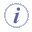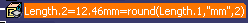# Mathematical Functions

Sample (illustrates interpolations): KwrInterpolations.CATPartNote that the input of the cosine function must be an angle despite its signature that allows real numbers. As a consequence, cos(3.14) for example is equivalent to cos (3.14 * 1rad) in the Formula editor. However a warning is displayed. Note that this applies also to the sinus and the tangent functions.

• abs(Real): Real
Calculates the absolute value of the argument.
• ceil(Real): Real
Returns the smallest integer value that is greater than or equal to the value specified in the argument.
• floor(Real):Real
Returns the largest integer value that is less than or equal to the value specified in the argument.
• int(Real):Real
Returns the integer part of the argument.
• min(Real,Real):Real, max(Real,Real)
Returns the minimum or maximum of a set of values specified in the argument.
• sqrt(Real):Real
Returns the square root.
• log(Real):Real
Returns the logarithm.
• ln(Real):Real
Returns the natural logarithm.
• round(Real):Real
Round rounds numbers of the form x.5 toward the nearest even integer.
• round(Real, String, Integer):Real
Returns a rounded number. This method is available for the types requiring units (Length, Angle, ...).
• Real is the real number that you want to round (for example 13.552).
• String is the unit (for example "mm").
• Integer corresponds to the number of digits that you want to display after the dot.A dimensioned value is required for the round function to work properly. Please find here below some examples on how the Round function works:
 round `(12.333mm,"mm",1)` will return 12.3 for the value is a length round `(12.333mm+1mm,"mm",1)` will display an error for the sum result is a non-dimensioned real value round `(-12.333mm,"mm",1)`will return an error for the subtraction result is a non-dimensioned real value The two last examples results using the + / - arithmetic operators may seem strange. However, this should be considered as a standard behavior. Beware that using the + / - arithmetic operators will always return a non-dimensioned value. When using this function, a parameter is logically selected as input so the + / - issue should not be recurrent in this context.
• exp(Real):Real
Returns the exponential.
• LinearInterpolation(arg1:Real, arg2:Real, arg3:Real) : Real
Must be used when creating a parallel curve from a law.
Example:
1 - Create a line in the Generative Shape Design workbench
2 - Access the Knowledge Advisor workbench and create the law below:
FormalReal.1 = LinearInterpolation(1,9,FormalReal.2)
3 - Back to the Generative Shape Design, create a parallel curve. Select the Law mode and specify the law above as the one to be applied.
• CubicInterpolation(arg1:Real, arg2:Real, arg3:Real) : Real
Must be used when creating a parallel curve from a law.
Example:
1 - Create a line in the Generative Shape Design workbench
2 - Access the Knowledge Advisor workbench and create the law below:
FormalReal.1 = CubicInterpolation(1,50,FormalReal.2)
3 - Back to the Generative Shape Design, create a parallel curve. Select the Law mode and specify the law above as the one to be applied.
• mod(Real,Integer): Real
Gives the remainder on division of Real by Integer. The returned value is of integer type.
Syntax: mod(Real,Integer):Real
Sample: Mod.CATPart
• Cos(Real):Real, cosh (Real): Real
Calculates the cosine(cos) or hyperbolic cosine(cosh).
Example
Real.1 = cos(45deg)
• tan(Real): Real, tanh(Real): Real
Calculates the tangent(tan) or hyperbolic tangent (tanh).
• sin(Real):Real, sinh(Real):Real
Calculates the sine or hyperbolic sine.
• asin(Real):Real, asinh(Real):Real
Calculates the arcsine or hyperbolic arcsine.
• acos(Real):Real, acosh(Real):Real
Calculates the arccosine or hyperbolic arccosine.
• atan(Real):Real, atanh(Real):Real
Calculates the arctangent or hyperbolic arctangent.
• max(arg1: Real, arg2: Real, ...):Real
Returns the highest parameter.
• min(arg1: Real, arg2: Real, ...):Real
Returns the smallest parameter.
• not(Boolean):Boolean
Verifies the contrary of the expression between parentheses.
Example: not(A==B) is the equivalent of A<>B.For these methods to be efficient, use real numbers only.